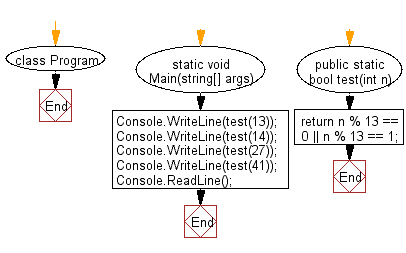﻿ C# - Check a non-negative number is a multiple of 13 or not# C# Sharp Basic Algorithm Exercises: Test whether a given non-negative number is a multiple of 13 or it is one more than a multiple of 13

## C# Sharp Basic Algorithm: Exercise-42 with Solution

Write a C# Sharp program to test whether a given non-negative number is a multiple of 13 or it is one more than a multiple of 13.

Sample Solution:-

C# Sharp Code:

``````using System;
using System.Linq;
namespace exercises
{
class Program
{
static void Main(string[] args)
{
Console.WriteLine(test(13));
Console.WriteLine(test(14));
Console.WriteLine(test(27));
Console.WriteLine(test(41));
}
public static bool test(int n)
{
return n % 13 == 0 || n % 13 == 1;
}
}
}
```
```

Sample Output:

```True
True
True
False```

Flowchart:C# Sharp Code Editor:

Improve this sample solution and post your code through Disqus

What is the difficulty level of this exercise?

Test your Programming skills with w3resource's quiz.

﻿